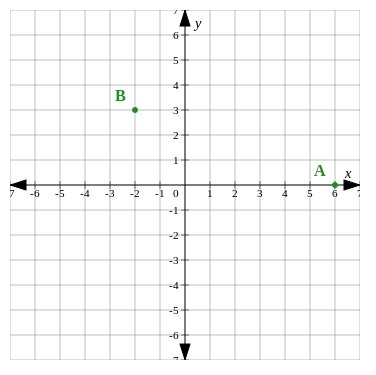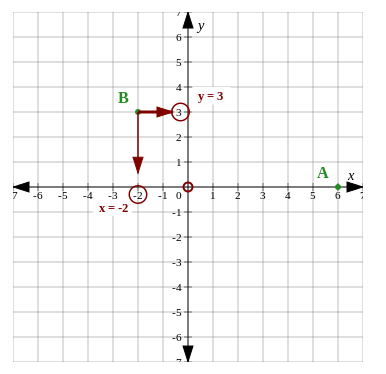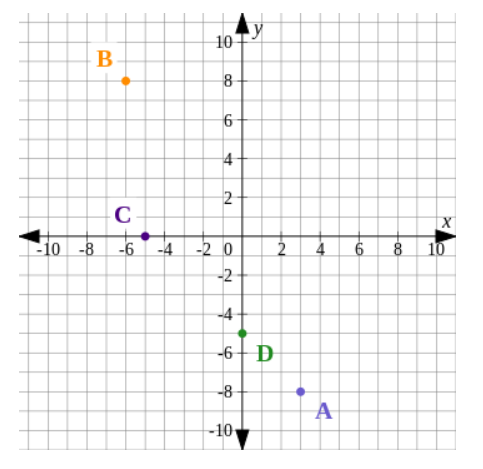Home Practice
For learners and parents For teachers and schools
Textbooks
Full catalogue
Pricing SupportLog in

We think you are located in United States. Is this correct?

# The Cartesian Plane

## 24.2 The Cartesian plane

In the previous chapter, we learned that the position of any point on the Cartesian plane can be represented by a set of coordinates called an ordered pair. An ordered pair consists of a $$T(4; 5)$$.

## Worked example 24.1: Finding the coordinates of a given point

The diagram shows the Cartesian plane and two points. The points are labelled $$A$$ and $$B$$. What are the coordinates of point $$B$$?### Read the coordinates from the axes.

We need to find the $$x$$-value and the $$y$$-value which tell us the position of point $$B$$ from the origin.

To find the $$x$$-value, we read how many units on the $$x$$-axis point $$B$$ is left or right of the origin (where the two axes cross; labelled 0). The $$x$$-coordinate is $$-2$$.

To find the $$y$$-value, we read how many units on the $$y$$-axis point $$B$$ is up or down from the origin. The $$y$$-coordinate is $$3$$.Notice that the value of the $$x$$-coordinate shows that the point lies to the left or to the right of the origin. The value of the $$y$$-coordinate shows that the point lies above or below the origin.

Remember the order of coordinate pairs: $$(x;y)$$. The $$x$$-coordinate is always written first and the $$y$$-coordinate is written second.

The coordinates of point $$B$$ are $$(-2;3)$$.

Exercise 24.1
1. Plot the four points $$P(-2; 5)$$, $$Q(-2; -2)$$, $$R(4; -2)$$ and $$S(4; 5)$$ on the Cartesian plane.

2. Name the quadrant in which each point lies.

3. What type of shape is $$PQRS$$?

1.2. $$P$$ lies in quadrant II $$Q$$ lies in quadrant III $$R$$ lies in quadrant IV $$S$$ lies in quadrant I
3. $$PQRS$$ is a rectangle.
1. Plot the points $$A(-4; 5)$$, $$B(-2; -3)$$ and $$C(0; 2)$$ on the Cartesian plane.

2. In which quadrant does point $$B$$ lie?

3. What type of shape is $$ABC$$?

1.2. Point $$B$$ lies in quadrant III
3. $$ABC$$ is a scalene triangle.
1. Plot the points $$M(0; -4)$$, $$N(6; -4)$$, $$P(6; 2)$$ and $$Q(0; 2)$$ on the Cartesian plane.

2. What type of shape is $$MNPQ$$?

1.2. $$MNPQ$$ is a square

Use the diagram to answer the questions.1. For which point does $$y = -8$$?
2. For which point(s) does $$x = -6$$?
3. For which point does $$y = -5$$?
4. For which point(s) does $$x = 0$$?
5. For which point(s) does $$y = 0$$?
6. Write down the coordinates of points $$A$$, $$B$$, $$C$$ and $$D$$.
1. $$A$$
2. $$B$$
3. $$D$$
4. $$D$$
5. $$C$$
6. $$A(3; -8)$$, $$B(-6; 8)$$, $$C(-5; 0)$$, $$D(0; -5)$$